# Mathematics - Signals And Systems - LTI Convolution Properties

in StemSocial2 months ago[Image1]

## Introduction

Hey it's a me again @drifter1!

Today we continue with my mathematics series about Signals and Systems in order to cover LTI Convolution Properties.

So, without further ado, let's get straight into it!

## LTI Convolution Properties

As we saw in the previous article of the series, one way to represent signals and systems is using a sum of weighted, delayed impulses. For Discrete-Time and in the case of linear time-invariant (LTI) systems, this convolution sum is defined as follows: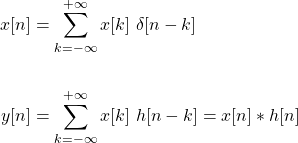In order to get into Continuous-Time signals and systems, its possible to approximate the result using combinations of weighted, delayed rectangular pulses. For increasingly narrow rectangular pulses, the representation then approaches the following integral, called a convolution integral: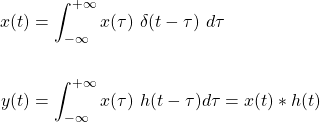When talking about LTI Systems, these convolution sums and integrals also satisfy these common properties of Algebra:

• Commutative
• Associative
• Distributive
Let's get into each of those with examples.

### Commutative Property

For LTI Systems, the response is the same when the input, x[n] or x(t), and impulse response, h[n] or h(t), are interchanged. This basically means that LTI systems can be put in series (or cascaded) in any order, as we saw already mentioned in the previous article.

Mathematically, the commutative property is expressed as follows: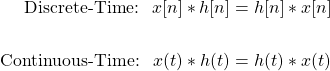#### Example

For example suppose a system with impulse response h, which takes an input x. The output of this system is equal to the convolution sum or integral x * h. But, because of commutativity the same output can also be taken by using h as an input and x as the impulse response.

Visually this can be interpreted more easily: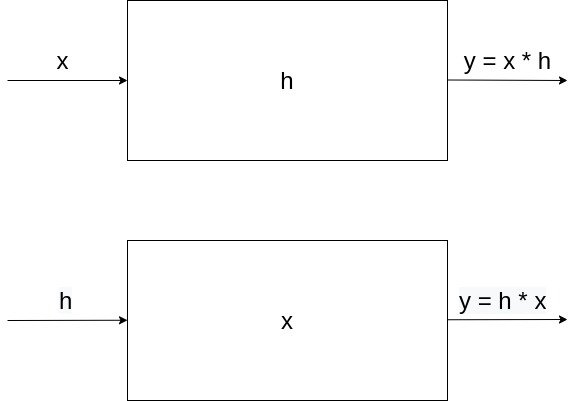[Custom Figure using draw.io]

### Associative Property

In addition to commutativity, there is also associativity. The convolution of LTI systems satisfies the associative property as the order at which the convolutions of multiple impulse responses h[n] or h(t) are calculated results in the same system response.

Mathematically, this basically means that the parantheses can be rearranged, which can be expressed as: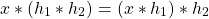So, it doesn't matter if the convolution for system 1 with impulse response h1 for input x is calculated first, or if the total impulse response of the two systems, 1 and 2, is calculated and then "associated" to the input x.

#### Example

Let's suppose two systems, system 1 and system 2, with impulse response h1 and h2 respectively. These two systems are cascaded for input x resulting into the output convolution x * h1 * h2. Due to assocativity, the order at which the convolutions are calculated doesn't matter, which means that the following three calculations and visual representations are feasible: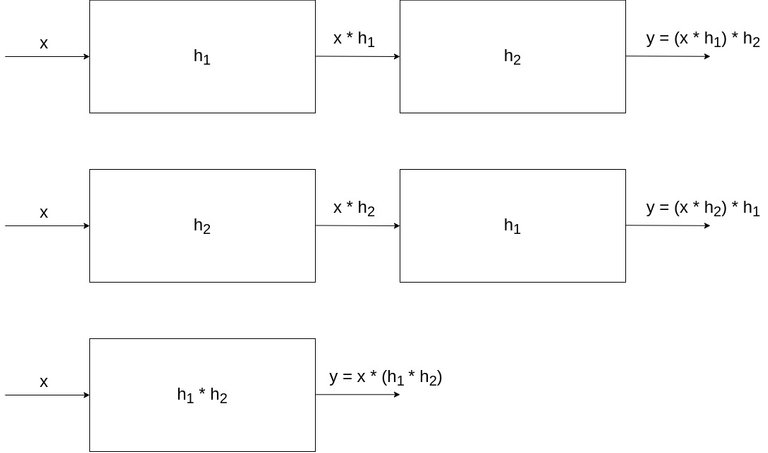[Custom Figure using draw.io]

### Distributive Property

Lastly, there is also the distributive property that applies to parallel interconnections of LTI systems. Due to distributivity, multiple parallel LTI systems that are summed together, can be easily collapsed into a single LTI system.

This basically means that calculating the response for some input x for two separate systems with impulse response h1 and h2 respectively and then summing those responses, is the same as giving the input x to a system with an impulse response that is equal to the sum of the individual impulse responses h1 + h2. Mathematically this can be interpreted as follows: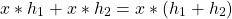#### Example

Suppose three parallel systems with impulse responses h1, h2 and h3, which all take in the same input x. The individual responses are summed together in order to form some bigger system. Due to distributivity its possible to just sum the respective impulse responses in order to calculate the system response.

Visually this can be understood much better.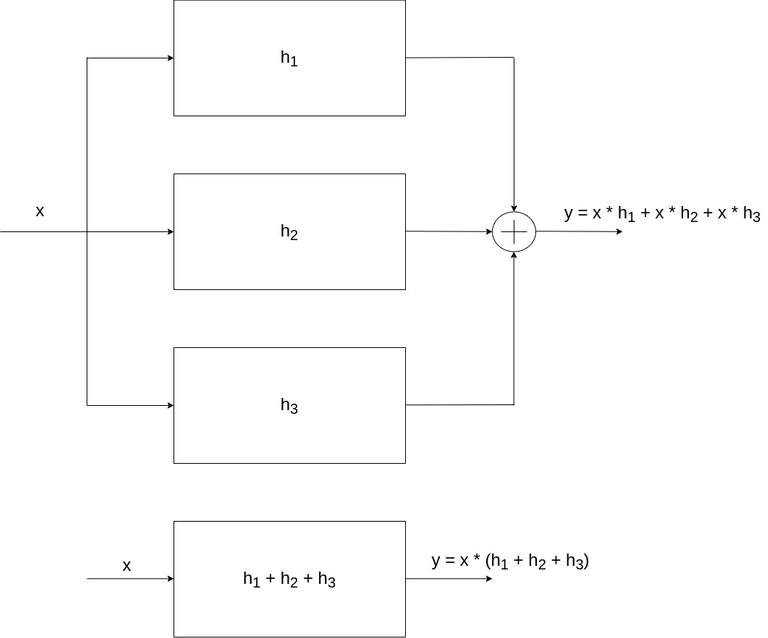[Custom Figure using draw.io]

## Final words | Next up

And this is actually it for today's post! Till next time!

See Ya!Keep on drifting!

Sort:

Convolution is vital in doing digital signal and image processing. We can use convolution to change continuous to discrete or in reverse.Thanks for your contribution to the STEMsocial community. Feel free to join us on discord to get to know the rest of us!

Please consider supporting our funding proposal, approving our witness (@stem.witness) or delegating to the @stemsocial account (for some ROI).

Please consider using the STEMsocial app app and including @stemsocial as a beneficiary to get a stronger support.